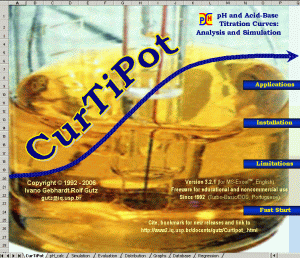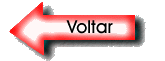Curvas de Titulação Potenciométrica e pHCurTiPot  para Excel® Programa gratuito (freeware) para simulação e análise de  Curvas de Titulação Potenciométrica, cálculos de pH e de equilíbrios ácido-base Presente no ensino-aprendizagem e na prática de titulações ácido-base desde 1992 Clique para obter cópia de CurTiPot no site do autor

Algumas Aplicações

•  Cálculos de pH de soluções aquosas simples ou complexas (até três dezenas de espécies ácidas e básicas);

•  Análise de dados de pH em função de volume de titulante (experimentais ou simulados);

»  representação gráfica das curvas, com derivadas e diagramas de distribuição das espécies;

»  localização precisa das inflexões das curvas (por interpolação com regressão com splines);

»  determinação quimiométrica (RNLMP) de concentrações e pKs (inclusive de amostras diluídas com múltiplos componentes);

•  Simulação de curvas de titulação ácido-base;

»  titulações simples, ácidos e bases multipróticos e misturas complexas;

»  simulação de dispersão (erros experimentais aleatórios) nas medidas de pH e de volume, para avaliar efeito nos resultados;

•  Geração de diagramas de protonação e de distribuição (composição fracionária) em função do pH.CurTiPot  for Excel® Freeware for simulation and analysis of Potentiometric Titration Curves and calculations of pH and acid-base equilibria   Since 1992 a hi-rated software for simulation and analysis of acid-base titration curves Go to the download page of CurTiPot

Some Applications

•  pH calculations of simple solutions and complex mixtures (>30 species)

of acids and bases in aqueous solutions;

•  pH vs. titrant volume data analysis of simulated and  real experimental titrations:

»  graphical representation of curves, derivatives and distribution of species;

»  precise evaluation of inflections of the curves by interpolation and smoothing with cubic splines;

»  determination of multiple concentrations and pKs by nonlinear regression, advantageous for diluted and complex samples;

•  Simulation of acid-base titration curves;

»  data generation of simple and complex mixtures of acids and bases (>30 species);

»  simulation of data dispersion (random experimental errors) in pH  and volume;

»  overlay of curves (>10) for visualization of the effect of changing parameters;

•  Generation of protonation diagrams and distribution curves of species vs. pH and vs. volume of titrant.